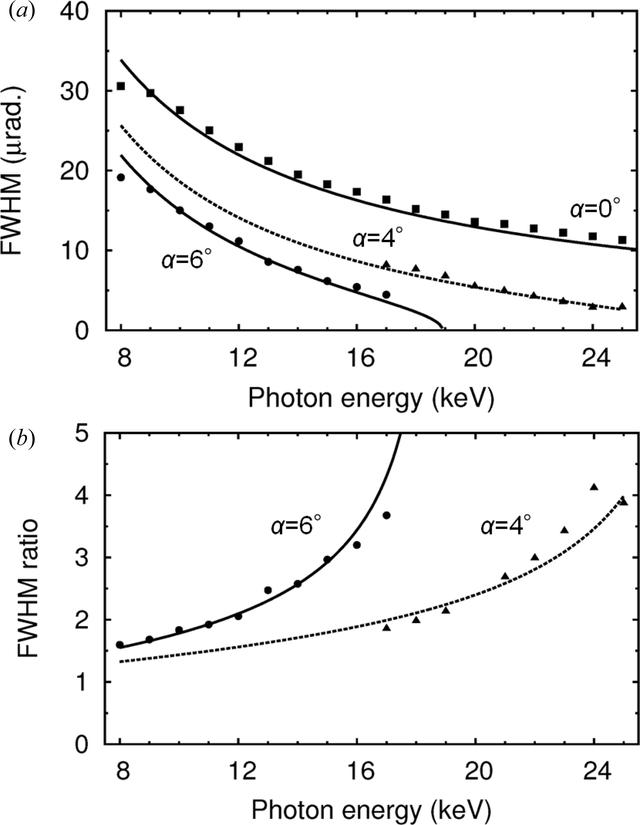disable zoom     view article Figure 6 (a) Intrinsic FWHMs of rocking curves of the first Si 111 crystal. (b) FWHM ratios of asymmetric geometries compared with the symmetric one. Filled squares, circles and triangles denote the experimental results in a symmetric double-crystal geometry (α = 0°) and in asymmetric geometries at α = 6° and 4°, respectively. Solid and dashed lines denote calculations.JOURNAL OFSYNCHROTRONRADIATION
ISSN: 1600-5775
Volume 26| Part 3| May 2019| Pages 750-755